﻿ UUV集群协同探测与数据融合技术研究
 舰船科学技术2017, Vol. 39Issue (12): 70-75PDF
UUV集群协同探测与数据融合技术研究

Research on UUVs cooperative detection and data fusion
ZHOU Hong-kun, GE Xi-yun, QIU Zhong-liang, Feng Xue-lei, CHEN Nan-ruo
State Key Laboratory of Deep-sea Manned Vehicles, China Ship Scientific Research Center, Wuxi 214082,China
Abstract: Principle and characteristic of unmanned underwater vehicles (UUVs) cooperative detection are described in this paper, and the developments and applications status abroad are introduced as well. A master-slave network based scheme for cooperative detection is proposed here, and the detection performances are analyzed according to three different formation patterns, and the data fusion approach integrating detection, navigation and communication is discussed.
Key words: UUV     cooperative detection     data fusion
0 引　言

UUV集群协同探测具有容错能力强、配置灵活、网络拓扑结构可动态调整的特点，可有效降低成本、扩大能力、提高效率和探测概率。UUV个体配备不同的任务模块，群体间通过资源共享弥补单UUV能力的不足，扩大执行任务范围；合理规划UUV集群探测路径，可以缩短作业时间，覆盖更大的工作区域；多艘UUV组成分布式、可灵活快速布放的水下探测网，可以多角度、多方位地提高远程目标探测概率与精度；UUV集群协作系统所具有的高度并行性和天然冗余性，提供了较强的容错能力，提高了系统的柔性和鲁棒性；多UUV相互配合，智能化程度高，获取信息量丰富，能够使水下战场更加透明，控制区域可向前推进上百公里

1 集群协同探测的分类

UUV集群协同探测的分类按声呐系统可分为协同主动探测和协同被动探测；按照目标种类可分为协同固定目标探测和协同移动目标探测；按网络结构可分为主从结构协同探测和并列结构协同探测；按协同探测时机可分为预先协同探测和临时协同探测

UUV协同探测固定目标与协同探测运动目标的区别在于UUV使用的探测设备和数量要求不同。在探测装备的选择方面与主/被动工作模式的选择相同，由于固定目标一般不向外发射物理场，因此应使用主动探测设备进行探测；UUV探测运动目标时可使用被动设备探测其辐射噪声，必要时使用主动探测设备对目标进行定位。在UUV的数量方面，由于固定目标没有位移，所以探测时需要的UUV数量相对较少，而探测运动目标时，由于目标位置的变化和UUV航程航速的限制，可能会需要数量较多的UUV才能完成任务。

UUV预先协同探测是指在UUV执行探测任务之前，利用一定的时间进行协同组织，安排各UUV的分工和协同方法。临时协同探测是指在UUV执行某一任务的过程中，根据情况的变化临时抽调某些UUV进行协同探测。显然，预先协同探测能够对探测任务进行详细分析，对协同计划进行周密的安排，而临时协同探测则能够对复杂的海洋环境做出及时的反应。

2 集群协同探测的应用现状

3 集群协同探测技术研究 3.1 方案设计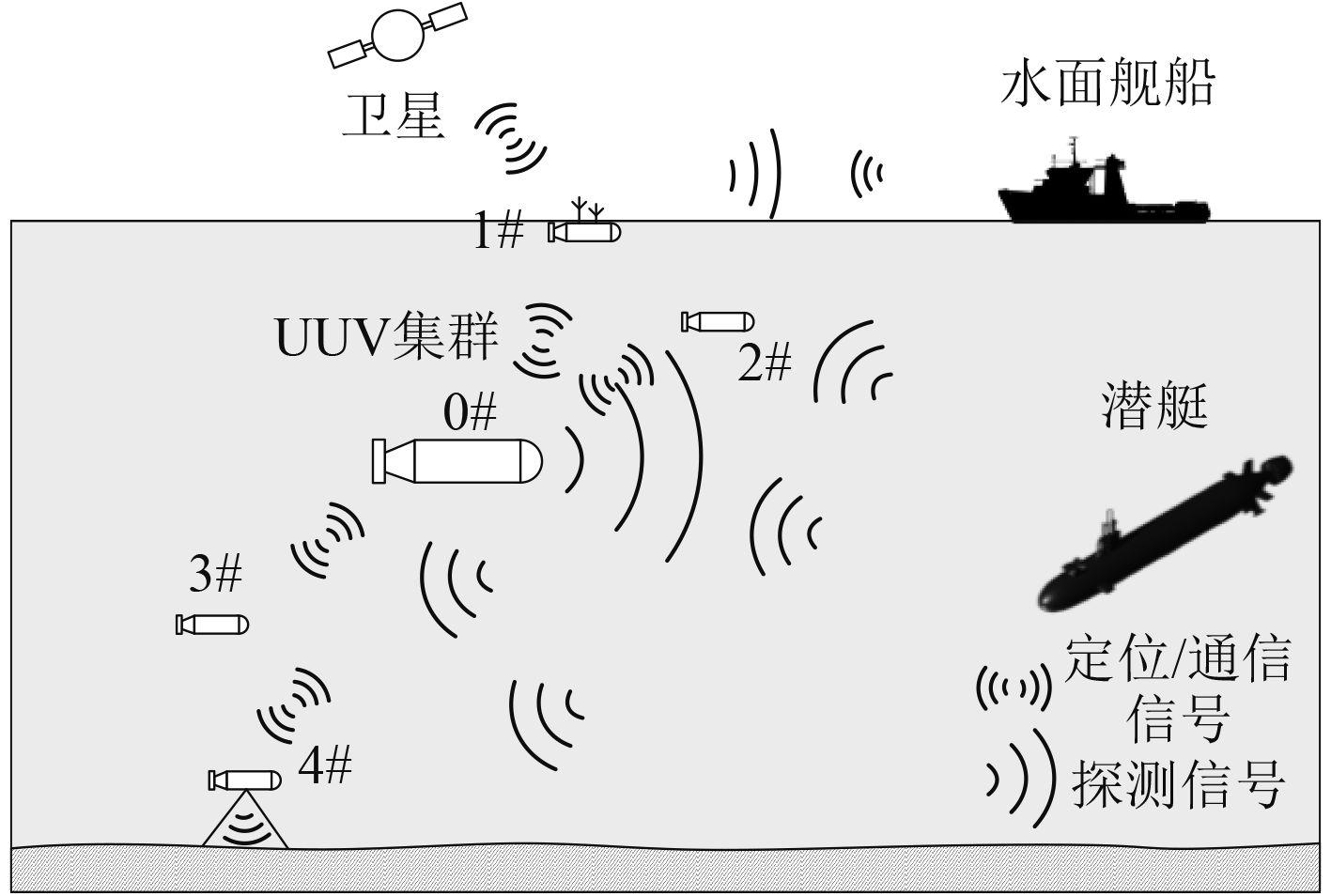图 1 UUV集群协同群探测示意图 Fig. 1 Schematic diagram of UUVs cooperative detection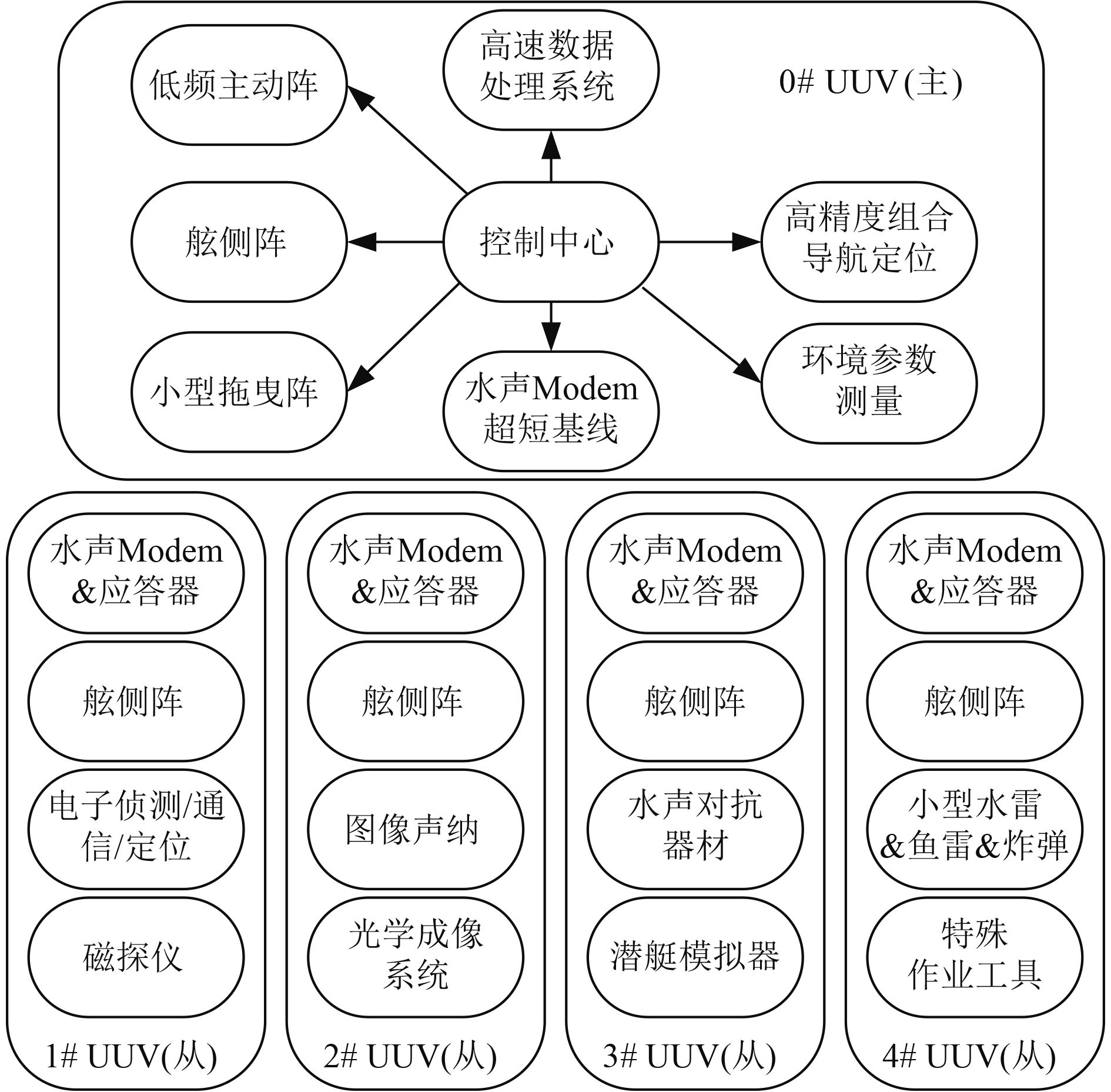图 2 UUV集群协同探测系统组成 Fig. 2 System configuration for UUVs cooperative detection

0#主节点配置智能控制中心，负责控制整个编队的运动和作业；配置高精度导航定位系统，为编队提供导航信息；配置大容量、高速数据处理系统，用于多传感器数据融合；搭载低频主动声呐、拖曳声呐，具备编队周围360°，100 km警戒搜索能力；配置大功率远/近程通信设备，用于对潜、对岸、对浮标、对UUV从节点、对水下固定节点的信息和数据传输；配备环境参数测量设备，用于海洋水体参数的测量。

1#从节点要求具备高航速的特点，可定时（或受命）上浮水面进行水面侦查、通信以及获取定位信息。主要配备电子侦测、通信和定位相关设备，用于对水面舰船的雷达与无线电信号的侦测、识别和通信，对低空飞机的雷达与无线电信号的侦测与通信，对卫星的通信和定位等；配备磁探仪用于水下磁异常探测。

2#从节点主要用于海底地形地貌测量、水雷探测、辅助地形匹配导航、辅助海底打捞等作业，主要装备声学成像系统、光学成像系统等。

3#从节点具备水声对抗能力，首先可用作为潜艇模拟器，利用主动声呐模拟水面、水下舰艇的自噪声，实现声学欺骗；其次是携带水声干扰器和气幕弹等装备，干扰敌方声呐工作。

4#从节点具备水下作业和攻击能力，携带作业工具用于布放/排除水雷，携带微型炸药用于引爆水雷，携带小型鱼雷用于攻击敌方水下航行器，配备特种工具用于破坏敌方UUV导航、通信、推进等系统，实现对敌方UUV、水下滑翔机、潜标的捕获。

3.2 水下警戒探测技术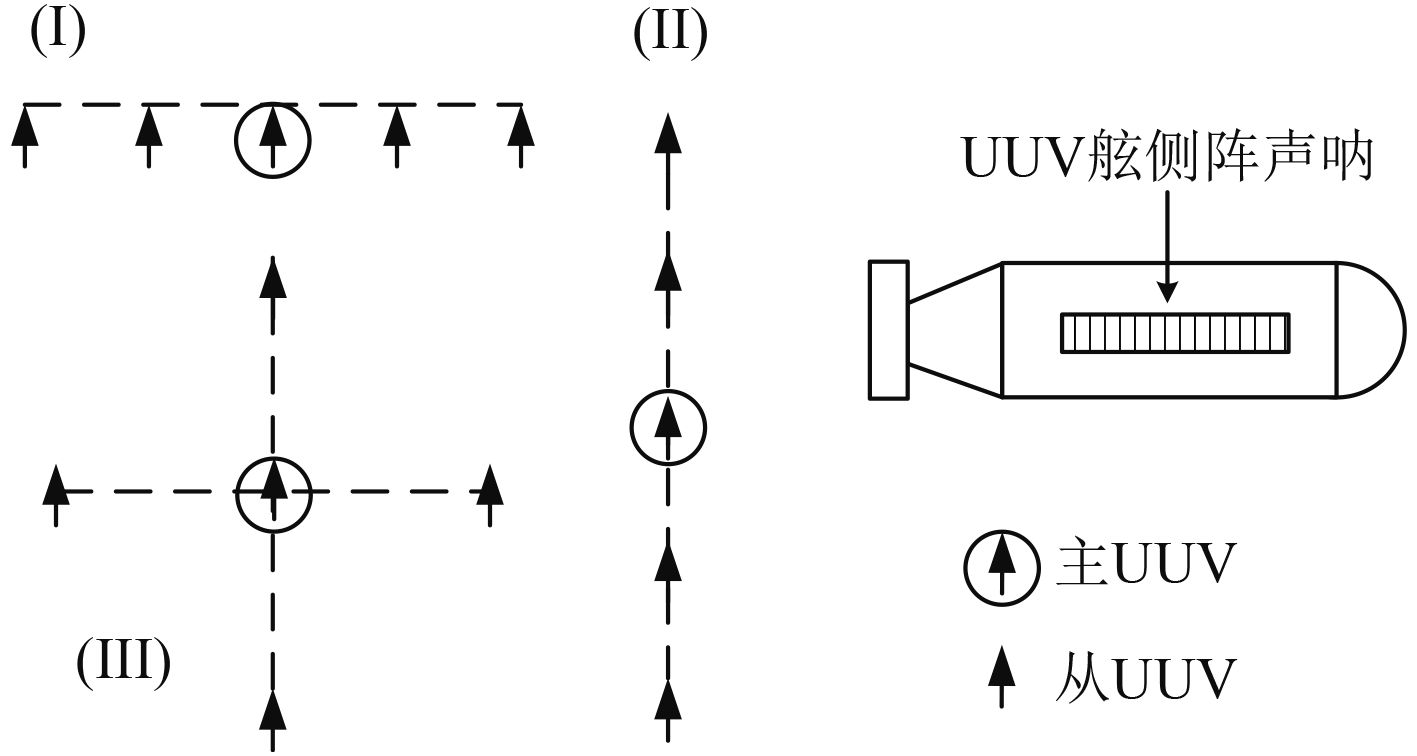图 3 UUV集群搜索三种队形结构 Fig. 3 Three formation patterns for UUVs search maneuver

1）一字横排型搜索

 ${D_1}\left( \theta \right) = \displaystyle\frac{{\sin \left[ {\displaystyle\frac{{{\rm{\pi }}L}}{\lambda }\sin \left( \theta \right)} \right]}}{{\displaystyle\frac{{{\rm{\pi }}L}}{\lambda }\left[ {\displaystyle\frac{{{\rm{\pi }}L}}{\lambda }\sin \left( \theta \right)} \right]}}\text{。}$ (1)

 ${D_2}\left( \theta \right) = \displaystyle\frac{{\sin \left[ {N\displaystyle\frac{{{\rm{\pi }}d}}{\lambda }\sin \left( {\theta - {\rm{\pi }}/2} \right)} \right]}}{{N\sin \left[ {\displaystyle\frac{{{\rm{\pi }}d}}{\lambda }\sin \left( {\theta - {\rm{\pi }}/2} \right)} \right]}} \text{。}$ (2)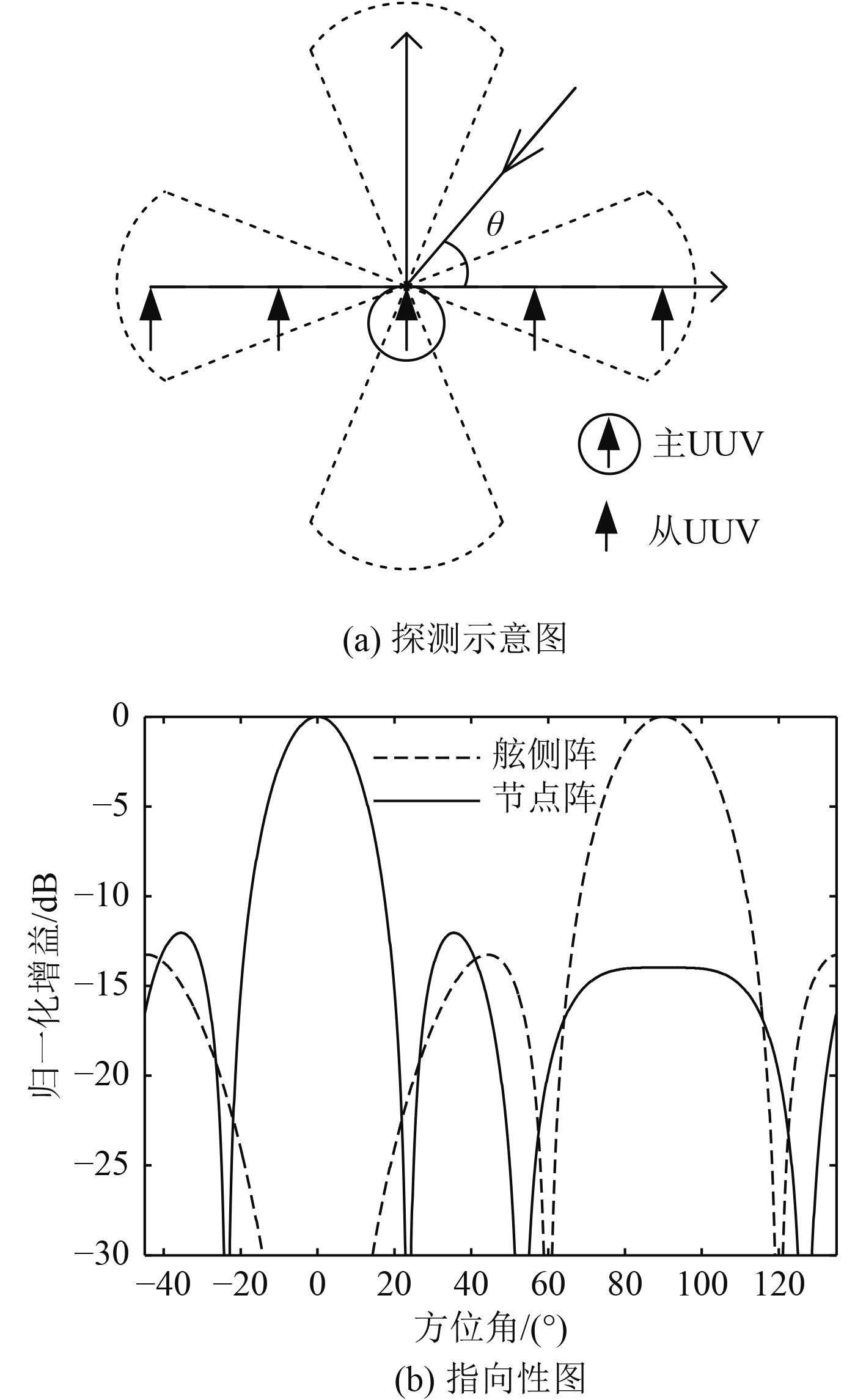图 4 队列I集群搜索 Fig. 4 Formation pattern I for UUVs search maneuver

2）一字纵排型搜索

 $\begin{array}{c}D\left( \theta \right) = {D_1}\left( \theta \right) \cdot {D_2}\left( \theta \right){\rm{ = }} \\ \displaystyle\frac{{\sin \left[ {\displaystyle\frac{{{\rm{\pi }}L}}{\lambda }\sin \left( \theta \right)} \right]}}{{\displaystyle\frac{{{\rm{\pi }}L}}{\lambda }\left[ {\displaystyle\frac{{{\rm{\pi }}L}}{\lambda }\sin \left( \theta \right)} \right]}} \cdot \displaystyle\frac{{\sin \left[ {N\displaystyle\frac{{{\rm{\pi }}d}}{\lambda }\sin \left( \theta \right)} \right]}}{{N\sin \left[ {\displaystyle\frac{{{\rm{\pi }}d}}{\lambda }\sin \left( \theta \right)} \right]}} \text{。}\end{array}$ (3)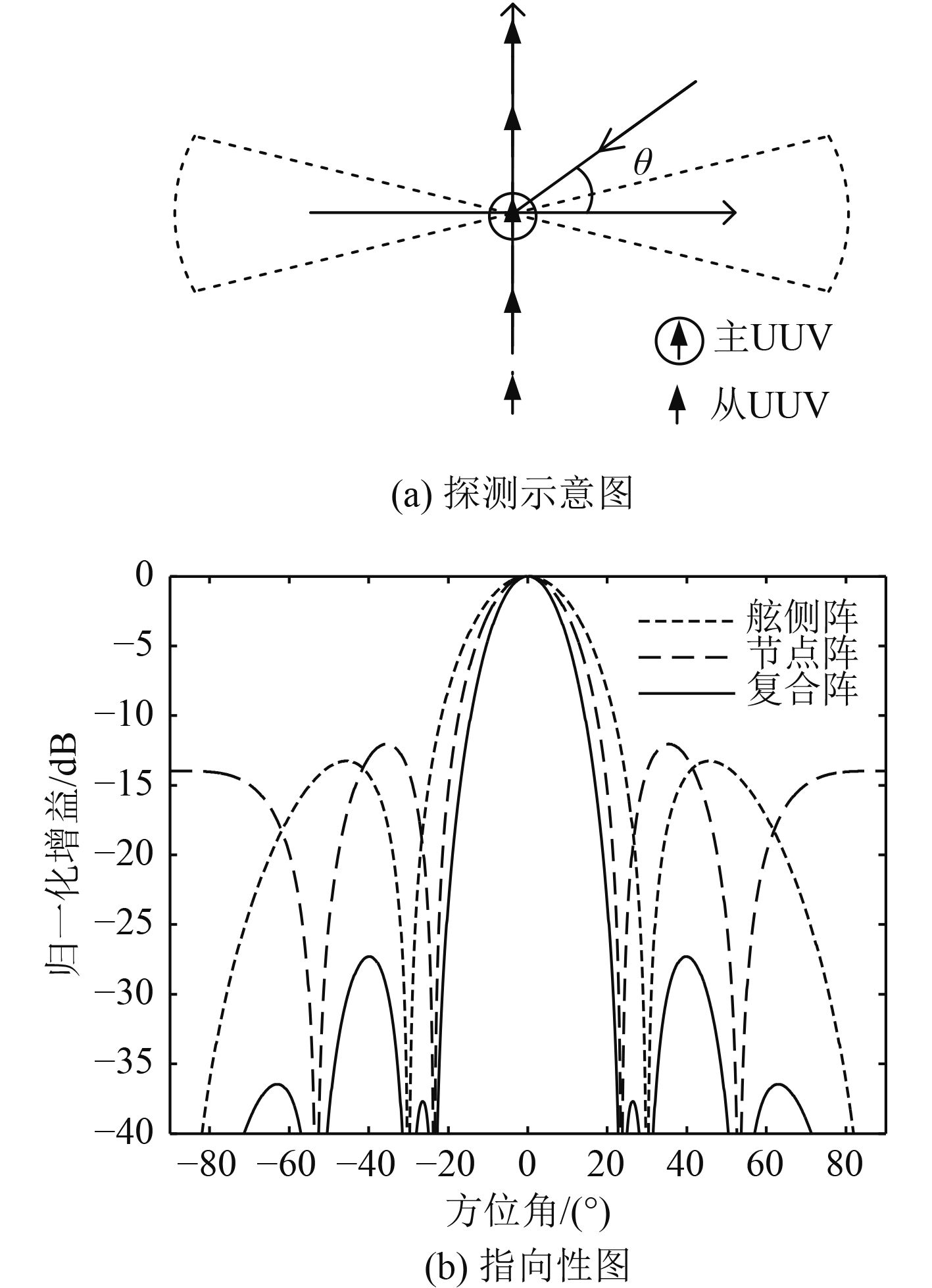图 5 队列II集群搜索 Fig. 5 Formation pattern II for UUVs search maneuver

3）十字交叉型搜索

 $\left\{ \begin{array}{l}{R^2} + 2Dc{\tau _1} - {\left( {c{\tau _1}} \right)^2} - 2RD\cos \varphi = 0 \text{，}\\{R^2} + 2Dc{\tau _2} - {\left( {c{\tau _2}} \right)^2} - 2RD\sin \varphi = 0 \text{，}\\{R^2} + 2Dc{\tau _3} - {\left( {c{\tau _3}} \right)^2} + 2RD\cos \varphi = 0 \text{，}\\{R^2} + 2Dc{\tau _4} - {\left( {c{\tau _4}} \right)^2} + 2RD\sin \varphi = 0 \text{。}\end{array} \right.$ (4)

 $\begin{array}{l}D = \left( {{c^2}\displaystyle\sum\limits_{i = 1}^4 {\tau _i^2} - 4{R^2}} \right)/\left( {2c\displaystyle\sum\limits_{i = 1}^4 {{\tau _i}} } \right),\;\\\tan \varphi = \displaystyle\frac{{\left( {{\tau _2} - {\tau _4}} \right)\left[ {2D - c\left( {{\tau _2} + {\tau _4}} \right)} \right]}}{{\left( {{\tau _1} - {\tau _3}} \right)\left[ {2D - c\left( {{\tau _1} + {\tau _3}} \right)} \right]}} \text{。}\end{array}$ (5)

 $\varphi = \arctan \frac{{\left( {{\tau _2} - {\tau _4}} \right)}}{{\left( {{\tau _1} - {\tau _3}} \right)}},{\sigma _\varphi } = \sqrt 2 \frac{c}{R}{\sigma _\tau } \text{。}$ (6)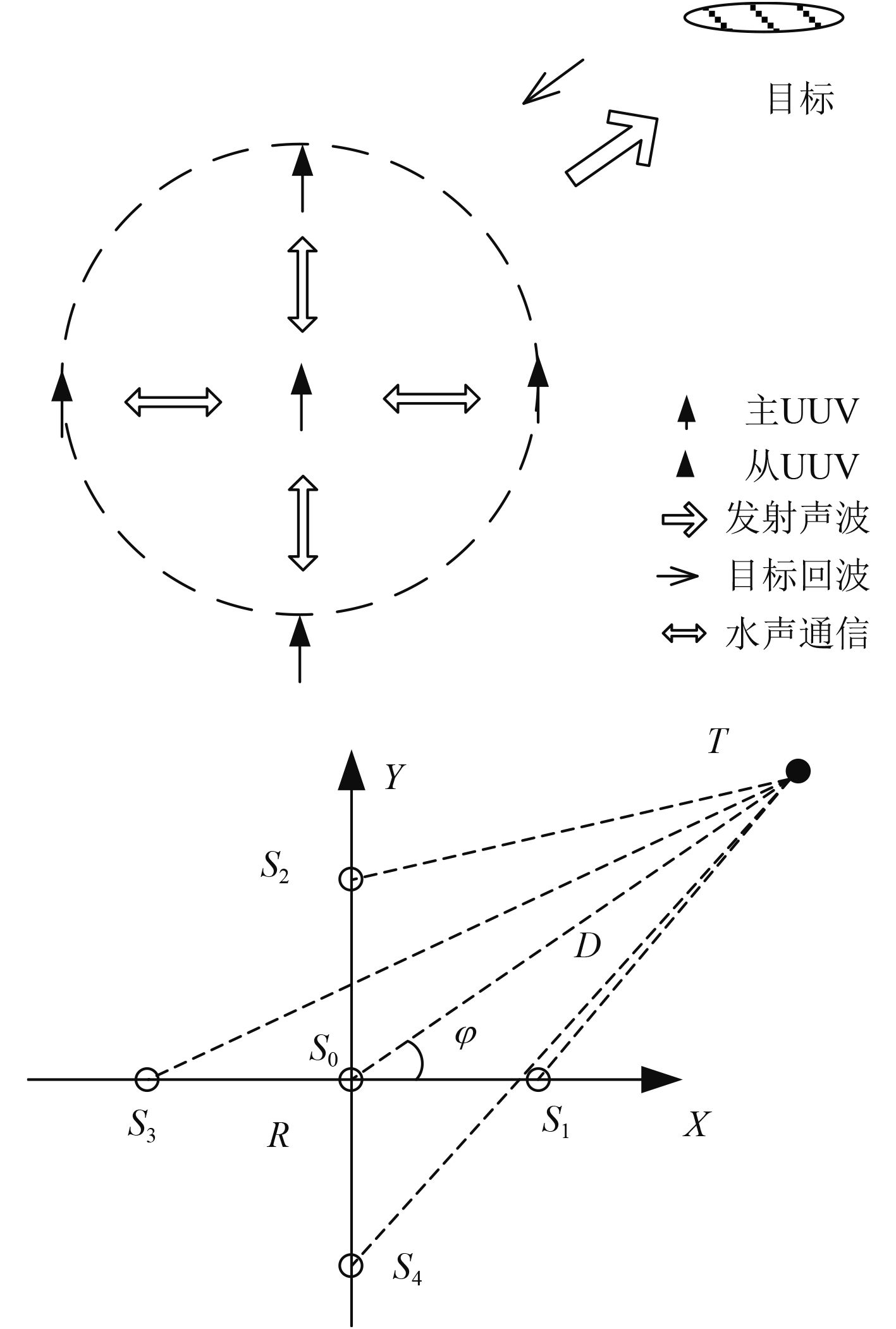图 6 队列III分布式探测示意图 Fig. 6 Schematic diagram of distributed detection for formation pattern III

4 数据融合技术

UUV之间的通信和定位问题是实现UUV协同探测的基础。UUV集群探测对各节点的相对位置要求比较高，由于主节点导航定位精度高，从节点导航定位能力弱，因此主节点需要利用水声定位系统（超短基线系统），对从节点进行定位，同时将位置信息、指令信息发送给从节点，这里水声定位与通信系统采用一体化设计。UUV主节点除利用自身的惯导设备进行导航以外，还可以利用其他UUV从节点获得的信号进行组合导航与定位。

1）UUV集群导航定位

2）水下战场信息收发

3）集群智能化探测与作业

4）故障决策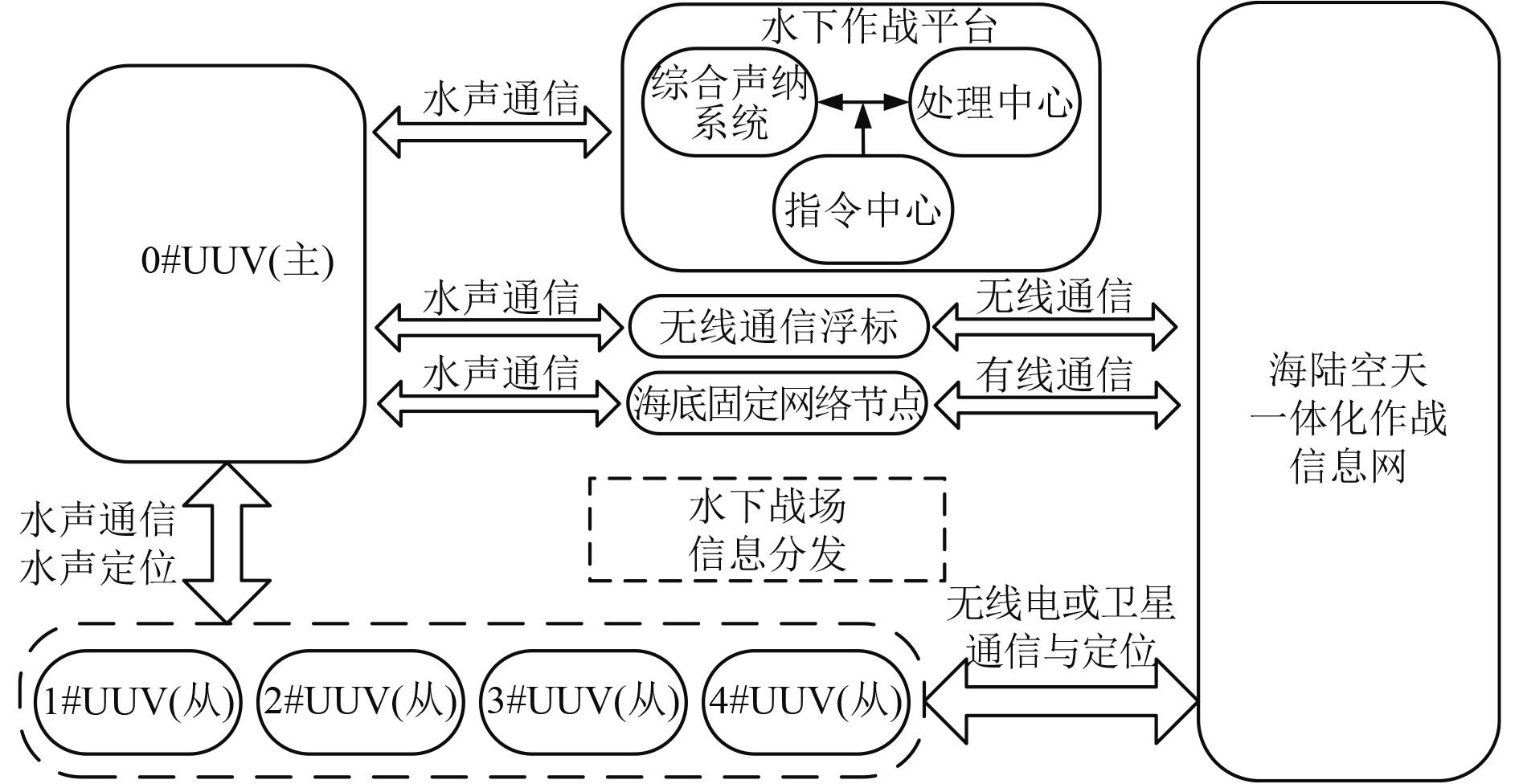图 7 UUV集群探测数据融合体系 Fig. 7 Data fusion system architecture for UUVs cooperative detection
5 结　语

  陈强. 水下无人航行器[M]. 北京: 国防工业出版社, 2014. CHEN Qiang. Unmanned underwater vehicle[M]. Beijing: National Defense Industry Press, 2014.  陈玮, 吴泽伟, 吴晓锋. UUV协同探测的现状与发展前景[J]. 舰船电子工程, 2009, 29 (2): 6–8. CHEN Wei, WU Ze-wei, WU Xiao-feng. Current status and future directions of uuvs cooperation probe[J]. Ship Electronic Engineering, 2009, 29 (2): 6–8.  单忠伟, 陈伏虎, 白兴宇. 基于UUV的水下警戒探测技术和发展趋势[J]. 声学与电子工程, 2009 (2): 45–48. SHAN Zhong-wei, CHEN Fu-hu, BAI Xing-yu. Underwater alert detection technology based on UUV and its development trend[J]. Acoustic and Electronics Engineering, 2009 (2): 45–48.  许真珍, 封锡盛. 多UUV协作系统的研究现状与发展[J]. 机器人, 2007, 29 (2): 186–192. XU Zhen-zhen, FENG Xi-shen. Current status and future directions of multiple UUV coopera-tion system[J]. Robot, 2007, 29 (2): 186–192.  BYRNE R H, ESKRIDGE S E, HURTADO J E, et a1. Algorithms and analysis for underwater vehicle plume tracing[R]. USA: DARPA, 2003.  李启虎. 声呐信号处理引论[M]. 北京: 科学出版社, 2012. LI Qi-hu. An introduction to SONAR signal pro-cessing[M]. Beijing: Science Press, 2012.  陈华伟, 赵俊渭, 郭业才. 五元十字阵被动声定位算法及其性能研究[J]. 探测与控制学报, 2003, 25 (4): 11–16. CHEN Hua-wei, ZHAO Jun-wei, GUO Ye-cai. Acoustic passive location algorithm based on a planar five-element array and its performance analysis[J]. Journal of Detention and Control, 2003, 25 (4): 11–16.